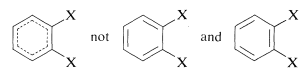# 3.6: Arenes

$$\newcommand{\vecs}{\overset { \rightharpoonup} {\mathbf{#1}} }$$ $$\newcommand{\vecd}{\overset{-\!-\!\rightharpoonup}{\vphantom{a}\smash {#1}}}$$$$\newcommand{\id}{\mathrm{id}}$$ $$\newcommand{\Span}{\mathrm{span}}$$ $$\newcommand{\kernel}{\mathrm{null}\,}$$ $$\newcommand{\range}{\mathrm{range}\,}$$ $$\newcommand{\RealPart}{\mathrm{Re}}$$ $$\newcommand{\ImaginaryPart}{\mathrm{Im}}$$ $$\newcommand{\Argument}{\mathrm{Arg}}$$ $$\newcommand{\norm}{\| #1 \|}$$ $$\newcommand{\inner}{\langle #1, #2 \rangle}$$ $$\newcommand{\Span}{\mathrm{span}}$$ $$\newcommand{\id}{\mathrm{id}}$$ $$\newcommand{\Span}{\mathrm{span}}$$ $$\newcommand{\kernel}{\mathrm{null}\,}$$ $$\newcommand{\range}{\mathrm{range}\,}$$ $$\newcommand{\RealPart}{\mathrm{Re}}$$ $$\newcommand{\ImaginaryPart}{\mathrm{Im}}$$ $$\newcommand{\Argument}{\mathrm{Arg}}$$ $$\newcommand{\norm}{\| #1 \|}$$ $$\newcommand{\inner}{\langle #1, #2 \rangle}$$ $$\newcommand{\Span}{\mathrm{span}}$$$$\newcommand{\AA}{\unicode[.8,0]{x212B}}$$

The so-called aromatic hydrocarbons, or arenes, are cyclic unsaturated compounds that have such strikingly different chemical properties from conjugated alkenes (polyenes) that it is convenient to consider them as a separate class of hydrocarbon. The simplest member is benzene, $$C_6H_6$$, which frequently is represented as a cyclic conjugated molecule of three single and three double carbon-carbon bonds. Actually, all the carbon-carbon bonds are equivalent (see Chapter 1) but it is convenient to represent the structure in the manner shown:A variety of substituted benzenes are known that have one or more of the hydrogen atoms of the ring replaced with other atoms or groups. In almost all of these compounds the special properties associated with the benzene nucleus are retained. A few examples of "benzenoid" hydrocarbons follow, and it will be noticed that the hydrocarbon substituents include alkyl, alkenyl, and alkynyl groups. Many have trivial names indicated in parentheses:The hydrocarbon group from benzene itself ($$C_6H_5-$$) is called a phenyl group and often is abbreviated as $$Ph$$ or less preferably by the symbol $$\phi$$. Generally, aryl groups are abbreviated as $$Ar$$, in contrast to alkyl groups for which we use $$R$$. Thus $$CH_3Ar$$ is a methyl-substituted arene, whereas $$RC_6H_5$$ is an alkyl-substituted benzene.

When there are two or more substituents on a benzene ring, position isomerism arises. Thus there are three possible isomeric disubstituted benzene derivatives according to whether the substituents have the 1,2, 1,3, or 1,4 relationship. The isomers commonly are designated as ortho, meta, and para (or o, m, and p) for the 1,2-, 1,3-, and 1,4-isomers, respectively. The actual symmetry of the benzene ring is such that only one 1,2-disubstitution product is found, despite the fact that two would be predicted if benzene had the 1,3,5-cyclohexatriene structure:When the benzene ring carries different substituents we shall cite them in alphabetical order (disregarding multiplying prefixes) and assign their positions on the ring with the lowest possible numbers. Examples areThe IUPAC nomenclature system for other types of compounds is given in Chapter 7 and is based on the fundamental rules described in this chapter.